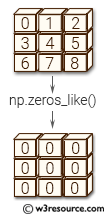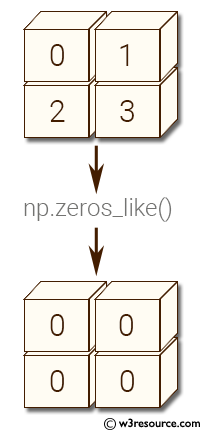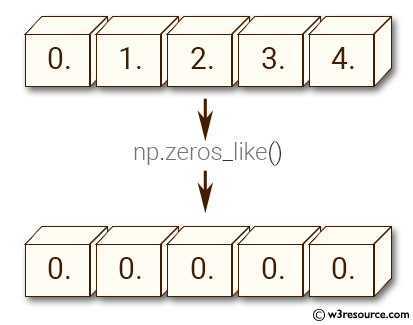# NumPy array creation: zeros_like() function

## zeros_like() function

The zeros_like() function is used to get an array of zeros with the same shape and type as a given array.

Syntax:

`numpy.zeros_like(a, dtype=None, order=’K’, subok=True)`Version: 1.15.0

Parameter:

Name Description Required /
Optional
a The shape and data-type of a define these same attributes of the returned array. Required
dtype Overrides the data type of the result. New in version 1.6.0. optional
order Overrides the memory layout of the result. 'C' means C-order, 'F' means F-order, 'A' means 'F' if a is Fortran contiguous, 'C' otherwise. 'K' means match the layout of a as closely as possible. New in version 1.6.0. optional
subok If True, then the newly created array will use the sub-class type of 'a', otherwise it will be a base-class array. Defaults to True. optional

Return value:

[ndarray] Array of zeros with the same shape and type as a.

Example: numpy.zeros()

``````>>> import numpy as np
>>> a = np.arange(4)
>>> a = a.reshape((2, 2))
>>> a
array([[0, 1],
[2, 3]])
>>> np.zeros_like(a)
array([[0, 0],
[0, 0]])
``````

Pictorial Presentation:Example: numpy.zeros() where data type is int

``````>>> import numpy as np
>>> b = np.arange(5, dtype=float)
>>> b
array([ 0.,  1.,  2.,  3.,  4.])
>>> np.zeros_like(b)
array([ 0.,  0.,  0.,  0.,  0.])
``````

Pictorial Presentation:Python - NumPy Code Editor:

Previous: zeros()
Next: full()

﻿